# DAV Class 5 Maths Chapter 7 Worksheet 9 Solutions

The DAV Maths Book Class 5 Solutions and DAV Class 5 Maths Chapter 7 Worksheet 9 Solutions of Multiplication and Division of Decimal Numbers offer comprehensive answers to textbook questions.

## DAV Class 5 Maths Ch 7 Worksheet 9 Solutions

Question 1.
Divide the following:
(a) 2.8 ÷ 0.7
Solution: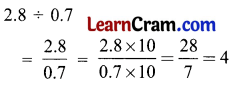(b) 3.6 ÷ 0.4
Solution: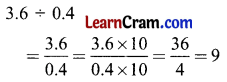(c) 3.2 ÷ 0.8
Solution:(d) 8.5 ÷ 1.7
Solution: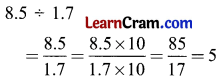(e) 0.75 ÷ 0.15
Solution:(f) 1.25 ÷ 2.5
Solution: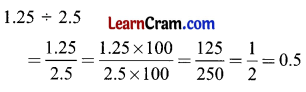(g) 5.6 ÷ 1.4
Solution: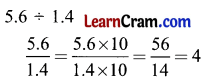(h) 1.44 ÷ 1.2
Solution: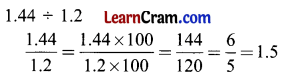(i) 0.993 ÷ 0.331
Solution: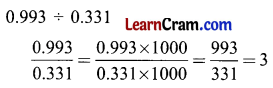(j) 25.925 ÷ 0.425
Solution: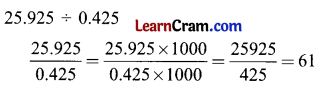DAV Class 5 Maths Chapter 7 Worksheet 9 Notes

Division of a Decimal Number by another Decimal Number

Example.
Divide 1.6 by 0.2
Solution:
Here dividend = 1.6, divisor = 0.2
1.6 ÷ 0.2 = $$\frac{1.6}{0.2}$$
(Division expressed as a fraction)
Let us change the divisor into a whole number.
$$\frac{1.6}{0.2}=\frac{1.6 \times 10}{0.2 \times 10}$$
= $$\frac{16}{2}$$
= 8
0.2 has one decimal place so, multiply the numerator and denominator by 10 to get an equivalent fraction.